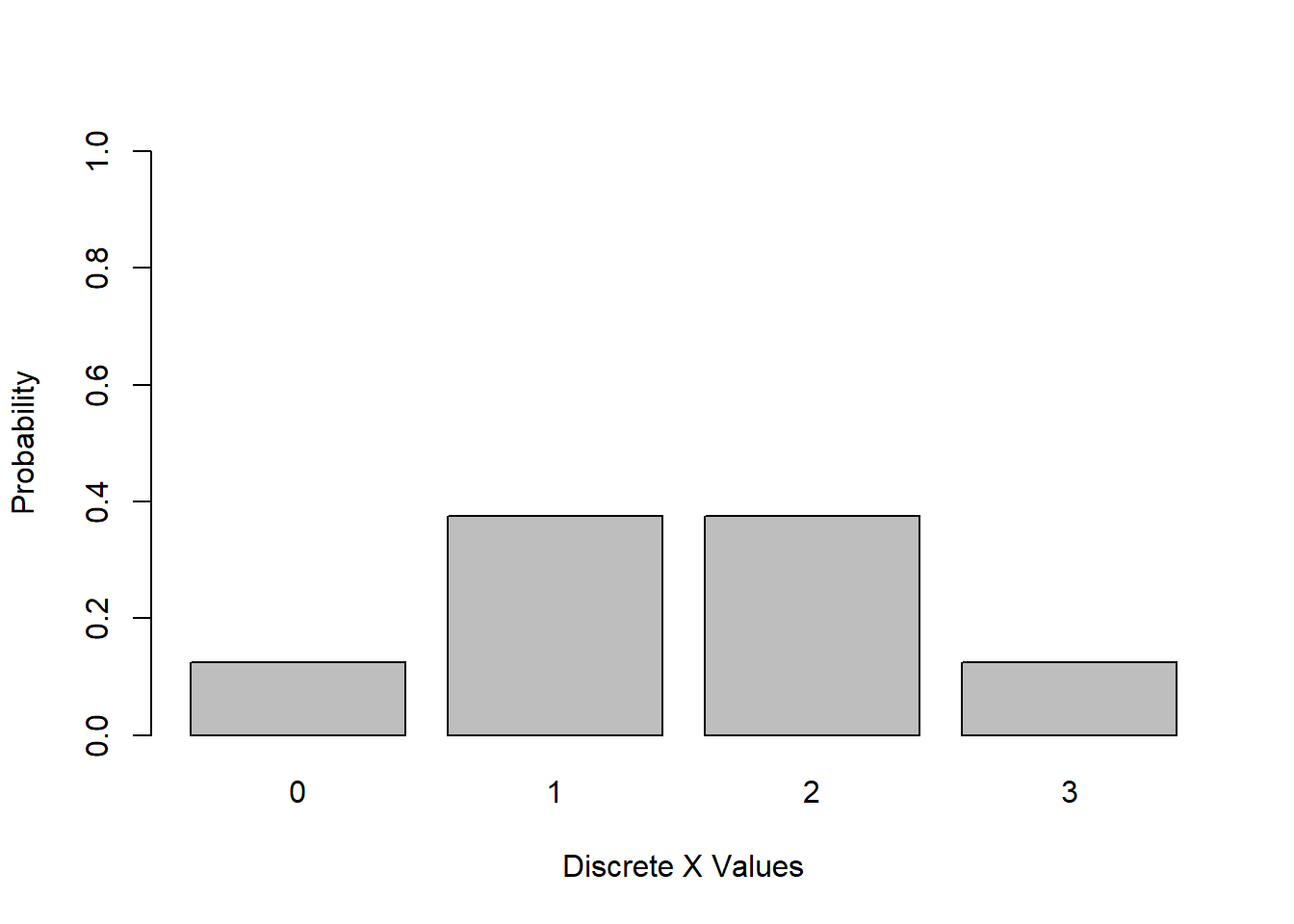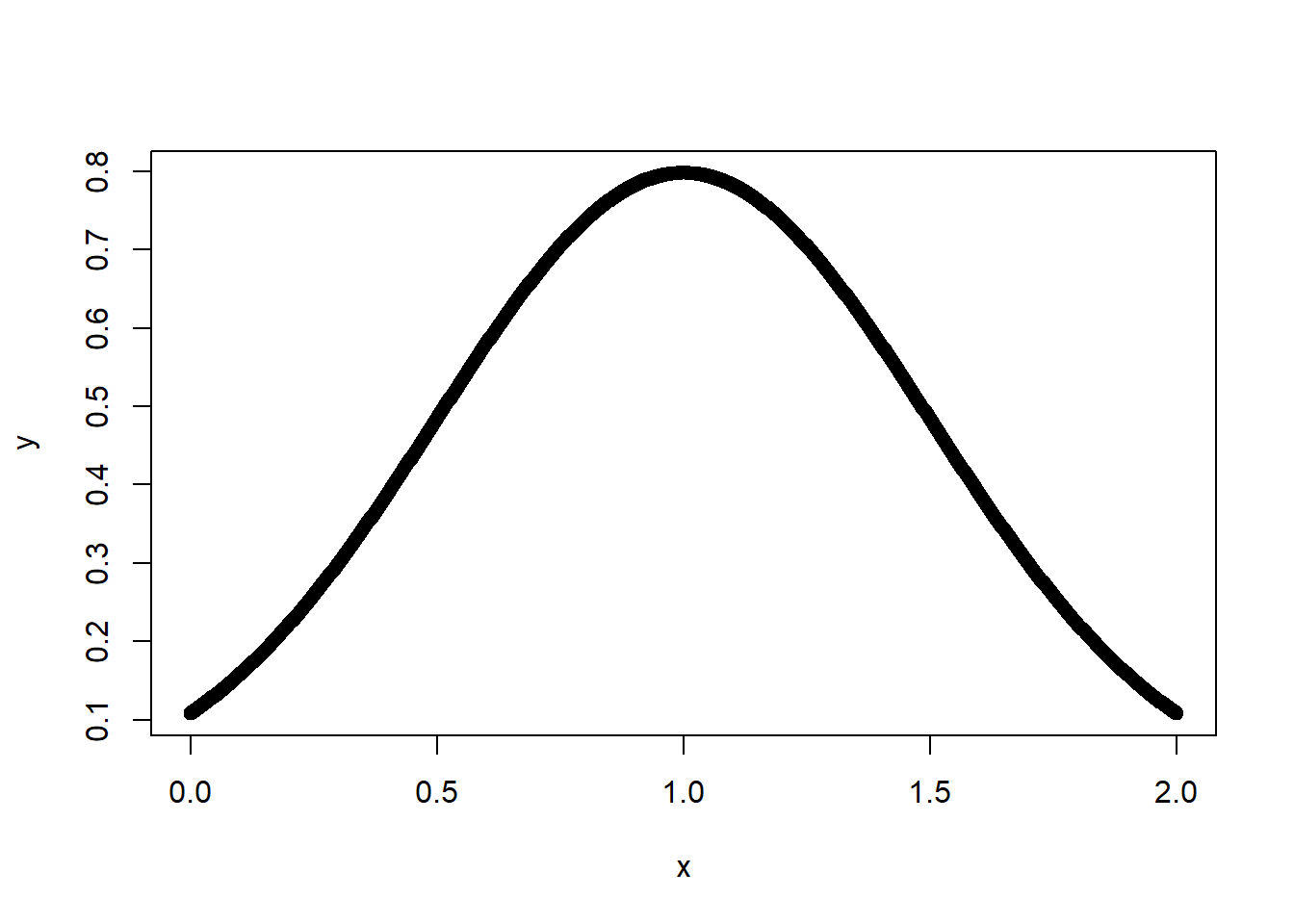Discrete Vs Continuous Random Variables in R

## Discrete Random Variable

Random in statistics does not mean ‘haphazard’ but it means a kind of order that emerges in the long run. For example we term unpredictable things that happen in our life as ‘random’. But we rarely see enough repetition of the same random phenomenon to observe a long term regulatrity that probability describes about ’random’ness.

If we define X={number of heads in a three coin tosses}, then X can take ONLY values 0,1,2 and 3. Hence X is a discrete variable. And since the coin tosses resulting in Heads can occur randomly in any order, X is a DISCRETE random variable.

``````x <- c(0,1,2,3)
y <- c(1/8,3/8,3/8,1/8)
barplot(y,names.arg=x,ylab = "Probability",  xlab="Discrete X Values", ylim = c(0,1))``````## continuous Random Variable (X)

However, if we define X ={all values between 0 and 2}, there can be infinitely many possible values, so X is called a continuous random variable. This is demonstrated below.

``````x <- seq(0,2,length=1000)
y <- dnorm(x, mean = 1, sd = 0.5)
plot(x,y)``````